# Methods and formulas for Multivariate EWMA Chart

Select the method or formula of your choice.

## Methods and formulas for MEWMA statistic for Multivariate EWMA chart

where:

Zi, the MEWMA vector, represents the weighting of previous subgroups.

where:

Xi, the subgroup mean vector, =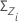= the weighted covariance matrix

where:

S is the sample covariance matrix. For more information on calculating the S matrix, go to Tsquared plotted point.

### Notation

TermDescription
nsubgroup size
Ssample covariance matrix. For more information on calculating the S matrix, see T-squared statistic

## Methods and formulas for control limits for Multivariate EWMA chart

The program used to calculate the upper control limit for the MEWMA chart is described in Bodden et al.1

## Methods and formulas for Box-Cox

### Box-Cox formula

If you use a Box-Cox transformation, Minitab transforms the original data values (Yi) according to the following formula:

where λ is the parameter for the transformation. Minitab then creates a control chart of the transformed data values (Wi). To learn how Minitab chooses the optimal value for λ, go to Methods and formulas for Box-Cox Transformation.

### Common λ values

The following table shows some commonly used λ values and their transformations.
λ Transformation
2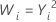0.5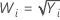0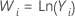−0.5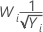−1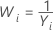1 K M. Bodden and S E. Rigdon (1999). A Program for Approximating the In-Control ARL for the MEWMA Chart. Journal of Quality Technology, 31,January, 120−123.
By using this site you agree to the use of cookies for analytics and personalized content.  Read our policy